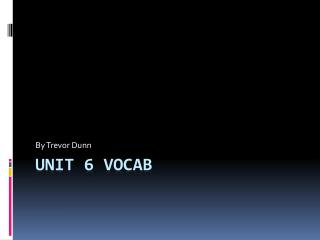DownloadDownload PresentationUnit 6 vocab

# Unit 6 vocab

Télécharger la présentation## Unit 6 vocab

- - - - - - - - - - - - - - - - - - - - - - - - - - - E N D - - - - - - - - - - - - - - - - - - - - - - - - - - -
##### Presentation Transcript

1. By Trevor Dunn Unit 6 vocab

2. Common denominator • A common denominator is when you multiply two or more fractions and you look at the denominator and see what numbers that are in the same groups so you can get the same denominator. • Here is a example of common denominators 2/3 and ½ 2/3*3 =4/6 ½*3=3/6 there both common denominator

3. Frequency table • A frequency table is a chart on which data tallied to find a frequency. Here is a example.

4. Great span • The Great span is the distance of the tip thumb to the tip pinkie when it is all stretched out.

5. Landmark • Is a data set in were it usually has the mean, mode, median ,maximum minimum and range. mean

6. Line plot • A line plot is a line where you put in data. • Pieces of candy kids eat in a day. 1 2 3 4 5 6 7 8 9 10

7. Maximum • The maximum is the greatest number. Here is a example of the maximum 1, 5, 7, 9, 9 is the maximum.

8. Median • The median is the middle number. Here is a example of the median 1, 3, 5, 8, 10, 5 is the median.

9. Minimum • The Minimum is the smallest number. For example 2, 5, 7, 9, 10 2 is the Minimum

10. Mode • The mode is the most often number. Here is a example 5, 8, 6, 7, 9, 8, 8, 3, 4, 2. 8 is the mode.

11. Quick common denominator • A quick common denominator is you just multiple the denominators. For example ¼* 2/3= 12 or just do 4*3=12

12. Stem and leaf plot • A stem and leaf plot is a why to put in data. Here is a example. Here is the data 11 32 58 67 1 1 3 2 5 8 6 7

13. Simplest form • A Simplest form is when you have a fraction that you can only divide by 1 with the numerator and denominator. Here is a example. 1/3

14. Thanks for watching • Bye